$$\require{cancel}$$

# B16: Magnetic Field: More Effects

The electric field and the magnetic field are not the same thing. An electric dipole with positive charge on one end and negative charge on the other is not the same thing as a magnetic dipole having a north and a south pole. More specifically: An object can have positive charge but it can’t have “northness”.

On the other hand, electricity and magnetism are not unrelated. In fact, under certain circumstances, a magnetic field will exert a force on a charged particle that has no magnetic dipole moment. Here we consider the effect of a magnetic field on such a charged particle.

FACT: A magnetic field exerts no force on a charged particle that is at rest in the magnetic field.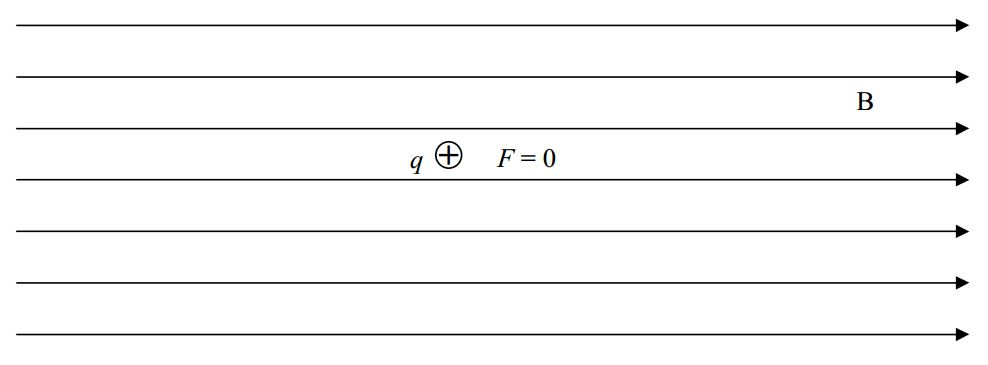FACT: A magnetic field exerts no force on a charged particle that is moving along the line along which the magnetic field, at the location of the particle, lies.FACT: A magnetic field does exert a force on a charged particle that is in the magnetic field, and, is moving, as long as the velocity of the particle is not along the line, along which, the magnetic field is directed. The force in such a case is given by:

$\vec{F}=q\vec{v}\times \vec{B}\label{16-1}$

Note that the cross product yields a vector that is perpendicular to each of the multiplicands. Thus the force exerted on a moving charged particle by the magnetic field within which it finds itself, is always perpendicular to both its own velocity, and the magnetic field vector at the particle’s location.

Consider a positively-charged particle moving with velocity $$v$$ at angle $$\theta$$ in the $$x-y$$ plane of a Cartesian coordinate system in which there is a uniform magnetic field in the $$+x$$ direction.To get the magnitude of the cross product $$\vec{v}\times \vec{B}$$ that appears in $$\vec{F}=q\vec{v}\times \vec{B}$$ we are supposed to establish the angle that $$\vec{v}$$ and $$\vec{B}$$ make with each other when they are placed tail to tail. Then the magnitude $$|\vec{v}\times \vec{B}|$$ is just the absolute value of the product of the magnitudes of the vectors times the sine of the angle in between them. Let’s put the two vectors tail to tail and establish that angle. Note that the magnetic field as a whole is an infinite set of vectors in the $$+x$$ direction. So, of course, the magnetic field vector $$\vec{B}$$, at the location of the particle, is in the $$+x$$ direction.Clearly the angle between the two vectors is just the angle $$\theta$$ that was specified in the problem. Hence,

$|\vec{v}\times \vec{B}|=|vB\sin \theta|,$

so, starting with our given expression for $$\vec{F}$$, we have:

$\vec{F}=q\vec{v}\times \vec{B}$

$|\vec{F}|=|q\vec{v} \times \vec{B}|$

$|\vec{F}|=|qvB\sin\theta|$

Okay, now let’s talk about the direction of $$\vec{F}=q\vec{v}\times \vec{B}$$. We get the direction of $$\vec{v}\times \vec{B}$$ and then we think. The charge $$q$$ is a scalar. If $$q$$ is positive, then, when we multiply the vector $$\vec{v}\times \vec{B}$$ by $$q$$ (to get $$\vec{F}$$ ), we get a vector in the same direction as that of $$\vec{v}\times \vec{B}$$. So, whatever we get (using the right-hand rule for the cross product) for the direction of $$\vec{v}\times \vec{B}$$ is the direction of $$\vec{F}=q\vec{v}\times \vec{B}$$. But, if $$q$$ is negative, then, when we multiply the vector $$\vec{v}\times \vec{B}$$ by $$q$$ (to get $$\vec{F}$$), we get a vector in opposite direction to that of $$\vec{v}\times \vec{B}$$. So, once we get the direction of $$\vec{v}\times \vec{B}$$ by means of the righthand rule for the cross product of two vectors, we have to realize that (because the charge is negative) the direction of $$\vec{F}=q\vec{v}\times \vec{B}$$ is opposite the direction that we found for $$\vec{v}\times \vec{B}$$.

Let’s do it. To get the direction of the cross product vector $$\vec{v}\times \vec{B}$$ (which appears in $$\vec{F}=q\vec{v}\times \vec{B}$$, draw the vectors $$\vec{v}$$ and $$\vec{B}$$ tail to tail.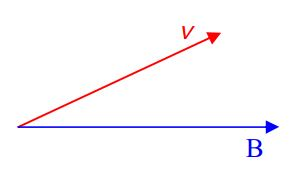Extend the fingers of your right hand so that they are pointing directly away from your right elbow. Extend your thumb so that it is at right angles to your fingers.Now, keeping your fingers aligned with your forearm, align your fingers with the first vector appearing in the cross product $$\vec{v}\times \vec{B}$$, namely $$\vec{v}$$.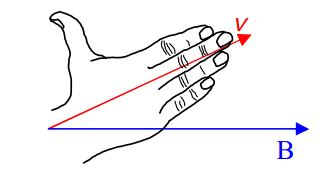Now rotate your hand, as necessary, about an imaginary axis extending along your forearm and along your middle finger, until your hand is oriented such that, if you were to close your fingers, they would point in the direction of the second vector.The direction in which your right thumb is now pointing is the direction of $$\vec{v}\times \vec{B}$$. We depict a vector in that direction by means of an $$\times$$ with a circle around it. That symbol is supposed to represent the tail feathers of an arrow that is pointing away from you.Let’s not forget about that $$q$$ in the expression $$\vec{F}=q\vec{v}\times \vec{B}$$. In the case at hand, the charged particle under consideration is positive. In other words $$q$$ is positive. So, $$\vec{F}=q\vec{v}\times \vec{B}$$ is in the same direction as $$\vec{v}\times \vec{B}$$.A magnetic field will also interact with a current-carrying conductor. We focus our attention on the case of a straight current-carrying wire segment in a magnetic field:

FACT: Given a straight, current carrying conductor in a magnetic field, the magnetic field exerts no force on the wire segment if the wire segment lies along the line along which the magnetic field is directed. (Note: The circuit used to cause the current in the wire must exist, but, is not shown in the following diagram.)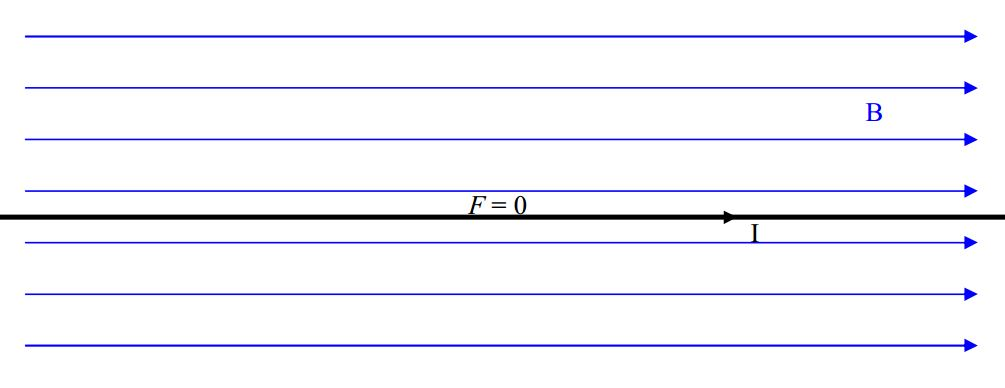FACT: A magnetic field exerts a force on a current-carrying wire segment that is in the magnetic field, as long as the wire is not collinear with the magnetic field.The force exerted on a straight current-carrying wire segment, by the (uniform) magnetic field in which the wire is located, is given by

$\vec{F}=I\vec{L}\times \vec{B}\label{16-2}$

where:

$$\vec{F}$$ is the force exerted on the wire-segment-with-current by the magnetic field the wire is in,

$$I$$ is the current in the wire,

$$\vec{L}$$ is a vector whose magnitude is the length of that segment of the wire which is actually in the magnetic field, and, whose direction is the direction of the current (which depends both on how the wire segment is oriented and how it is connected in the (not-shown) circuit.)

$$\vec{B}$$ is the magnetic field vector. The magnetic field must be uniform along the entire length of the wire for this formula to apply, so, $$\vec{B}$$ is the magnetic field vector at each and every point along the length of the wire.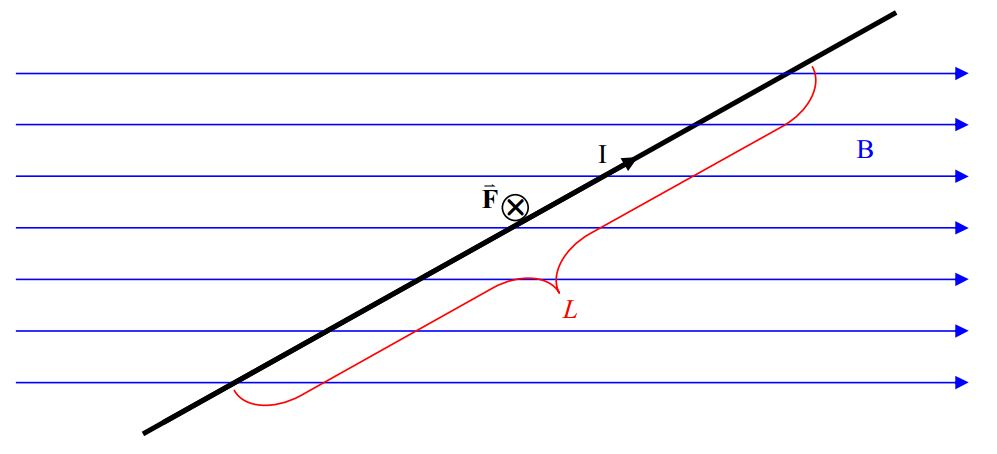Note that, in the preceding diagram, $$\vec{F}$$ is directed into the page as determined from $$\vec{F}=I\vec{L}\times \vec{B}$$ by means of the right-hand rule for the cross product of two vectors.

## Effect of a Uniform Magnetic Field on a Current Loop

Consider a rectangular loop of wire. Suppose the loop to be in a uniform magnetic field as depicted in the following diagram:Note that, to keep things simple, we are not showing the circuitry that causes the current in the loop and we are not showing the cause of the magnetic field. Also, the magnetic field exists throughout the region of space in which the loop finds itself. We have not shown the full extent
of either the magnetic field lines depicted, or, the magnetic field itself.

Each segment of the loop has a force exerted on it by the magnetic field the loop is in. Let’s consider the front and back segments first:Because both segments have the same length, both segments make the same angle with the same magnetic field, and both segments have the same current; the force $$\vec{F}=I\vec{L}\times \vec{B}$$ will be of the same magnitude in each. (If you write the magnitude as $$F=ILB\sin\theta$$, you know the magnitudes are the same as long as you know that for any angle $$\theta$$, $$\sin (\theta)=\sin (180^{\circ}-\theta)$$.) Using the right-hand rule for the cross product to get the direction, we find that each force is directed perpendicular to the segment upon which it acts, and, away from the center of the rectangle:The two forces, $$F_{\mbox{FRONT}}$$ and $$F_{\mbox{BACK}}$$ are equal in magnitude, collinear, and opposite in direction. About the only effect they could have would be to stretch the loop. Assuming the material of the loop is rigid enough not to stretch, the net effect of the two forces is no effect at all. So, we can forget about them and focus our attention on the left and right segments in the diagram.

Both the left segment and the right segment are at right angles to the magnetic field. They are also of the same length and carry the same current. For each, the magnitude of $$\vec{F}=I\vec{L}\times \vec{B}$$ is just $$IwB$$ where $$w$$ is the width of the loop and hence the length of both the left segment and the right segment.Using the right-hand rule for the cross product of two vectors, applied to the expression $$\vec{F}=I\vec{L}\times \vec{B}$$ for the force exerted on a wire segment by a magnetic field, we find that the force $$F=IwB$$ on the right segment is upward and the force $$F=IwB$$ on the left segment is downward.The two forces are equal (both have magnitude $$F=IwB$$ ) and opposite in direction, but, they are not collinear. As such, they will exert a net torque on the loop. We can calculate the torque about the central axis:by extending the lines of action of the forces and identifying the moment arms: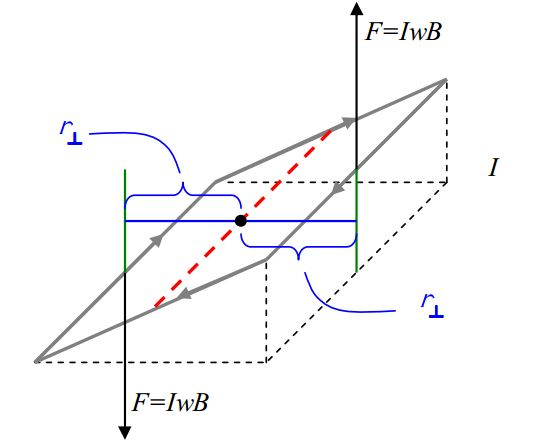The torque provided by each force is $$r_{\perp}F$$. Both torques are counterclockwise as viewed in the diagram. Since they are both in the same direction, the magnitude of the sum of the torques is just the sum of the magnitudes of the two torques, meaning that the magnitude of the total torque is just $$\tau=2r_{\perp}F$$. We can get an expression for $$2r_{\perp}$$ by recognizing, in the diagram, that $$2r_{\perp}$$ is just the distance across the bottom of the triangle in the front of the diagram: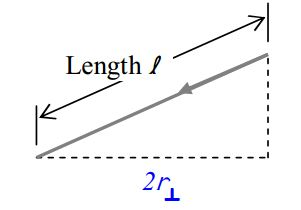and defining the angle $$\theta$$, in the diagram, to be the angle between the plane of the loop and the vertical.From the diagram, it is clear that $$2r_{\perp}=l \sin \theta$$.

Thus the magnetic field exerts a torque of magnitude

$\tau=r_{\perp} F$

$\tau=[l(\sin\theta)](IwB)$

on the current loop.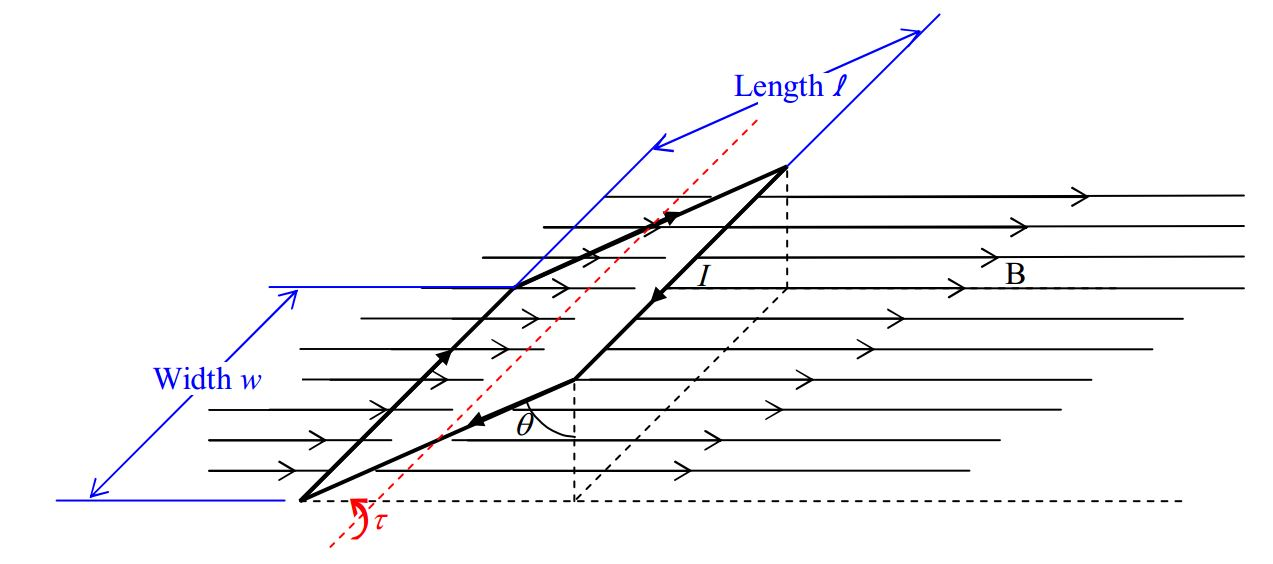The expression for the torque can be written more concisely by first reordering the multiplicands so that the expression appears as

$\tau=IlwB \sin \theta$

and then recognizing that the product $$lw$$ is just the area $$A$$ of the loop. Replacing $$lw$$ with $$A$$ yields:

$\tau=I\space AB \sin\theta$

Torque is something that has direction, and, you might recognize that $$\sin\theta$$ appearing in the preceding expression as something that can result from a cross product. Indeed, if we define an area vector to have a magnitude equal to the area of the loop,

$|\vec{A}|=lw$

and, a direction perpendicular to the plane of the loop,we can write the torque as a cross product. First note that the area vector as I have defined it in words to this point, could point in the exact opposite direction to the one depicted in the diagram. If, however, we additionally stipulate that the area vector is directed in accord with the righthand rule for something curly something straight, with the loop current being the something curly and the area vector the something straight (and we do so stipulate), then the direction of the area vector is uniquely determined to be the direction depicted in the diagram.

Now, if we slide that area vector over to the right front corner of the loop,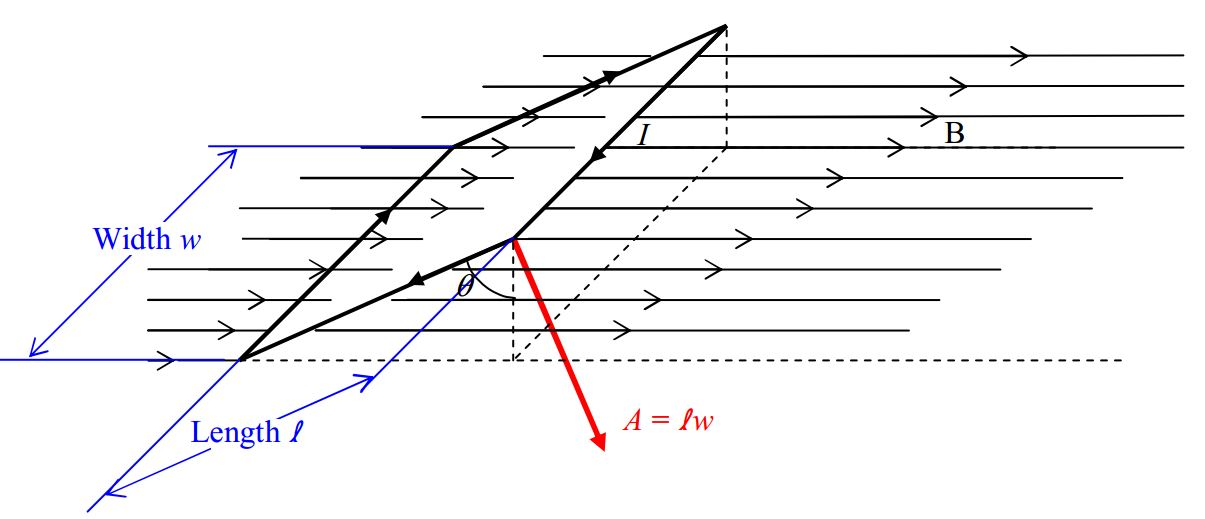it becomes more evident (you may have already noticed it) that the angle between the area vector $$\vec{A}$$ and the magnetic field vector $$\vec{B}$$, is the same $$\theta$$ defined earlier and depicted in the diagram just above.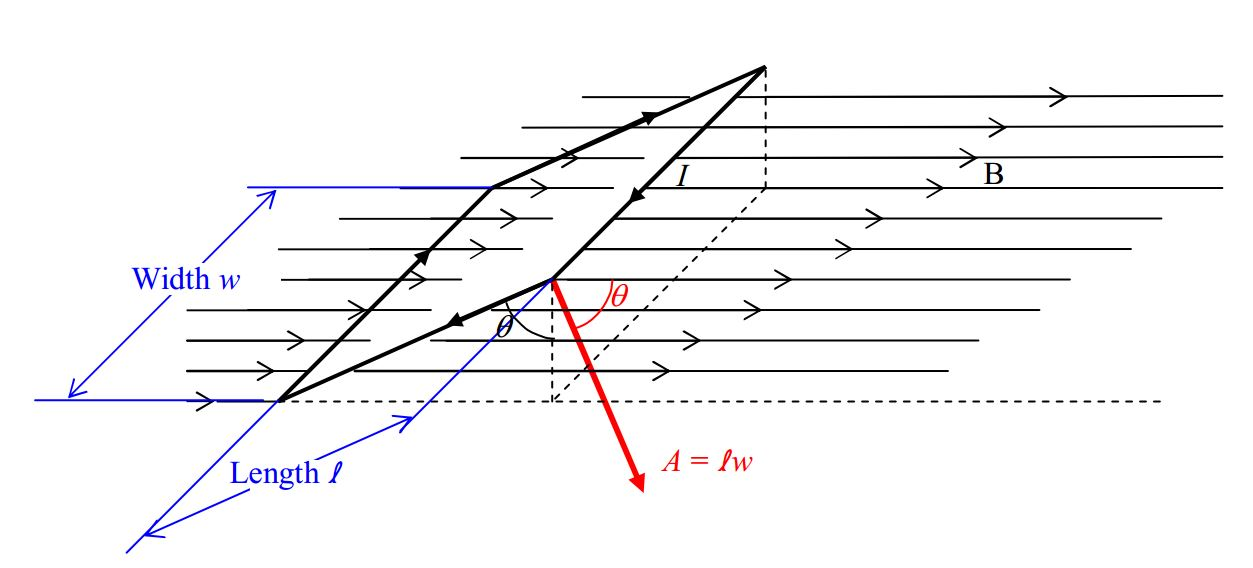This allows us to write our expression for the torque $$\tau=IAB \sin\theta$$ counterclockwise as viewed in the diagram, as:

$\vec{\tau}=I\vec{A}\times \vec{B}$

Check it out. The magnitude of the cross product $$|\vec{A}\times \vec{B}|$$ is just $$AB\sin \theta$$, meaning that our new expression yields the same magnitude $$\tau=I AB\sin \theta$$ for the torque as we had before. Furthermore, the right-hand rule for the cross product of two vectors yields the torque direction depicted in the following diagram.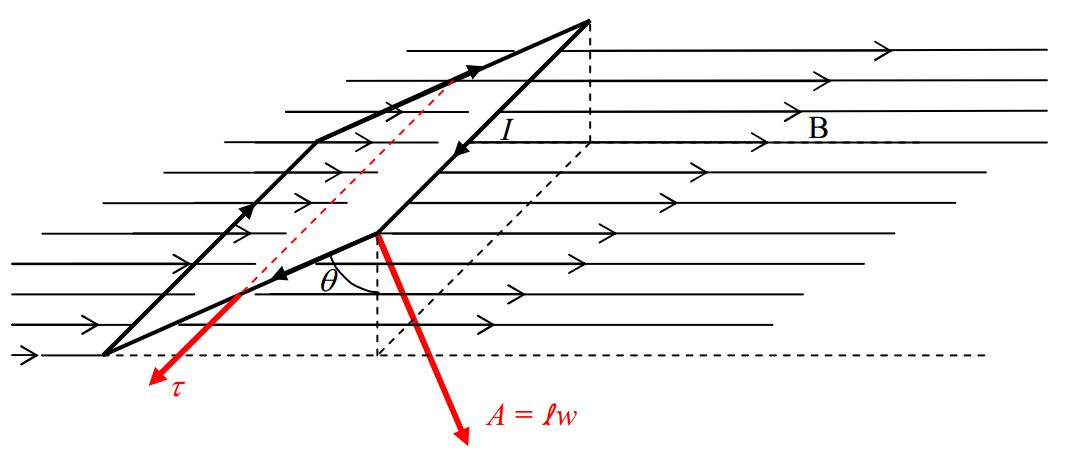Recalling that the sense of rotation associated with an axial vector is determined by the righthand rule for something curly, something straight; we point the thumb of our cupped right hand in the direction of the torque vector and note that our fingers curl around counterclockwise, as viewed in the diagram.Okay, we’re almost there. So far, we have the fact that if you put a loop of wire carrying a current $$I$$ in it, in a uniform magnetic field $$\vec{B}$$, with the loop oriented such that the area vector $$\vec{A}$$ of the current loop makes an angle $$\theta$$ with the magnetic field vector, then, the magnetic field exerts a torque

$\vec{\tau}=I\vec{A}\times \vec{B}$

on the loop.

This is identical to what happens to a magnetic dipole when you put it in a uniform magnetic field. It experiences a torque $$\vec{\tau}=\vec{\mu}\times \vec{B}$$. In fact, if we identify the product $$I\vec{A}$$ as the magnetic dipole moment of the current loop, then the expressions for the torque are completely identical:

$\vec{\tau}=\vec{\mu}\times \vec{B}\label{16-3}$

where:

$$\vec{\tau}$$ is the torque exerted on the victim. The victim can be either a particle that has an inherent magnetic dipole moment, or, a current loop.

$$\vec{\mu}$$ is the magnetic dipole moment of the victim. If the victim is a particle, $$\vec{\mu}$$ is simply the magnitude and direction of the inherent magnetic dipole moment of the particle. If the victim is a current loop, then $$\vec{\mu}=I\vec{A}$$ where $$I$$ is the current in the loop and $$\vec{A}$$ is the area vector of the loop, a vector whose magnitude is the area of the loop and whose direction is the direction in which your right thumb points when you curl the fingers of your right hand around the loop in the direction of the current. (See the discussion below for the case in which the victim is actually a coil of wire rather than a single loop.)

$$\vec{B}$$ is the magnetic field vector at the location of the victim.

A single loop of wire can be thought of as a coil of wire that is wrapped around once. If the wire is wrapped around $$N$$ times, rather than once, then the coil is said to have $$N$$ turns or $$N$$ windings. Each winding makes a contribution of $$I\vec{A}$$ to the magnetic dipole moment of the current loop. The contribution from all the loops is in one and the same direction. So, the magnetic moment of a current-carrying coil of wire is:

$\vec{\mu}=NI\vec{A}\label{16-4}$

where:

$$\vec{\mu}$$ is the magnetic moment of the coil of wire.

$$N$$ is the number of times the wire was wrapped around to form the coil. $$N$$ is called the number of windings. $$N$$ is also known as the number of turns.

$$I$$ is the current in the coil. The coil consists of one long wire wrapped around many times, so, there is only one current in the wire. We call that one current the current in the coil.

$$\vec{A}$$ is the area vector of the loop or coil. Its magnitude is the area of the plane shape whose perimeter is the loop or coil. Its direction is the direction your extended right thumb would point if you curled the fingers of your right hand around the loop in the direction of the current.

## Some Generalizations Regarding the Effect of a Uniform Magnetic Field on a Current Loop

We investigated the effect of a uniform magnetic field on a current loop. A magnetic field will exert a torque on a current loop whether or not the magnetic field is uniform. Since a current loop has some spatial extent (it is not a point particle), using a single value-plus-direction for $$\vec{B}$$ in $$\vec{\tau}=\vec{\mu} \times \vec{B}$$ will yield an approximation to the torque. It is a good approximation as long as the magnetic field is close to being uniform in the region of space occupied by the coil.

We investigated the case of a rectangular loop. The result for the torque exerted on the current-carrying loop or coil is valid for any plane loop or coil, whether it is circular, oval, or rectangular.